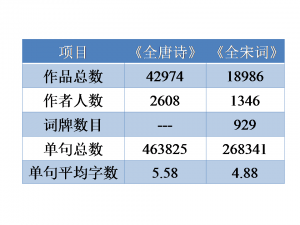4月 03

1.

```1 2 3 4 5 6 7 8 9 10 11 12 13 14 plot.tri <- function(n = 1000, col ="blue", ani=FALSE, cex=1.2){ p <- runif(n); X <- rbind(rep(0, n), rep(0, n)) B <- cbind(c(0,0),c(0.25,0.433),c(0.5,0)) if(ani) plot(0,0,xlim=c(0,1),ylim=c(0,0.85),type="n",xlab="",ylab="") for(i in 2:n){ pp <- p[i]; ind <- rank(c(c(1/3,2/3,1), pp), ties.method="min") X[,i] <- 0.5*X[,i-1] + B[,ind] if(ani) points(X[1,i], X[2,i],pch = ".", cex = 1, col = col) } if(!ani) plot(X[1,], X[2,],pch = ".", cex = cex, col = col, xlab="", ylab="") } plot.tri(100000, ani=TRUE)```
3月 30

在R中调用igraph绘制网络图

1. igraph简介

2. igraph is a free software package for creating and manipulating undirected and directed graphs. It includes implementations for classic graph theory problems like minimum spanning trees and network flow, and also implements algorithms for some recent network analysis methods, like community structure search.……【阅读全文】

3月 21

山人何处君不见？东风一花倚阑干！

二、数据

《全唐诗》及《全宋词》的原始数据均来自于网络。

三、工具

Shell命令行：转换编码（iconv）与换行符（fromdos）。
Perl（v5.10.1）：用来过滤、筛选原始数据，得到可以用于后续分析的格式化数据。
R（v2.12.2）：统计分析及图表绘制。

四、结果

1.基本信息3月 18

• 基本

一、数据管理

vector：向量
numeric：数值型向量
logical：逻辑型向量
character；字符型向量
list：列表
data.frame：数据框
c：连接为向量或列表
length：求长度
subset：求子集
seq，from:to，sequence：等差序列
rep：重复
NA：缺失值
NULL：空对象
sort，order，unique，rev：排序
unlist：展平列表
attr，attributes：对象属性
mode，typeof：对象存储模式与类型
names：对象的名字属性